# Data Processing with Pandas

In this article, we are going to see data manipulation using Python.

Pandas is a powerful, fast, and open-source library built on NumPy. It is used for data manipulation and real-world data analysis in python. Easy handling of missing data, Flexible reshaping and pivoting of data sets, and size mutability make pandas a great tool to perform data manipulation and handle the data efficiently.

Data Manipulation is changing data into a more organized format according to one’s requirement. Thus, Data Manipulation involves the processing of data into useful information. Through the pandas library data manipulation becomes easy. Hence, let’s understand Data Manipulation with Pandas in more detail. We will use Mall_Customers dataset to show the syntax of these functions in work as well.

## Python3

 `#Importing pandas library` `import` `pandas as pd`   `#Loading data into a DataFrame` `data_frame``=``pd.read_csv(``'Mall_Customers.csv'``)`

### Printing rows of the Data

By default, data_frame.head() displays the first five rows and data_frame.tail() displays last five rows. If we want to get first ‘n’ number of rows then we use, data_frame.head(n) similar is the syntax to print the last n rows of the data frame.

## Python3

 `#displaying first five rows` `display(data_frame.head())`   `#displaying last five rows` `display(data_frame.tail())`

Output: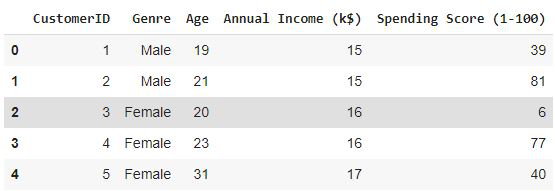First Five rows of the data frame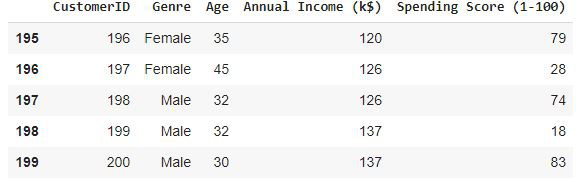Last Five rows of the data frame

## Python3

 `# Program to print all the column name of the dataframe` `print``(``list``(data_frame.columns))`

Output –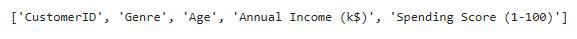### Summary of Data Frame

The functions info() prints the summary of a DataFrame that includes the data type of each column, RangeIndex (number of rows), columns, non-null values, and memory usage.

## Python3

 `data_frame.info()`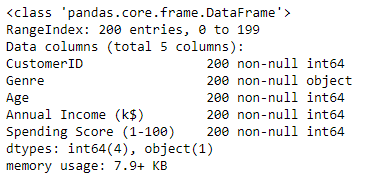Summary of the data frame

### Descriptive Statistical Measures of a DataFrame

The describe() function outputs descriptive statistics which include those that summarize the central tendency, dispersion, and shape of a dataset’s distribution, excluding NaN values. For numeric data, the result’s index will include count, mean, std, min, and max as well as lower, 50, and upper percentiles. For object data (e.g. strings), the result’s index will include count, unique, top, and freq.

## Python3

 `data_frame.describe()`

Output: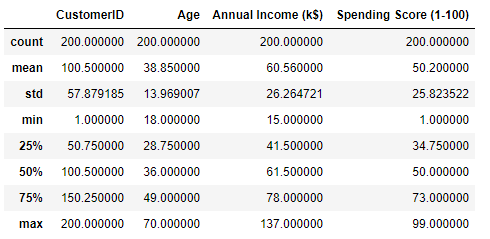Descriptive Statistical Measure of data frame

### Missing Data Handing

#### a) Find missing values in the dataset:

The isnull( ) detects the missing values and returns a boolean object indicating if the values are NA. The values which are none or empty get mapped to true values and not null values get mapped to false values.

## Python3

 `data_frame.isnull( )`

Output:

#### b) Find the number of missing values in the dataset:

To find out the number of missing values in the dataset, use data_frame.isnull( ).sum( ). In the below example, the dataset doesn’t contain any null values. Hence, each column’s output is 0.

## Python3

 `data_frame.isnull().``sum``()`

Output: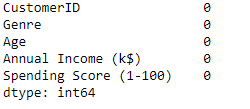Number of null values in each column

#### c) Removing missing values:

The data_frame.dropna( ) function removes columns or rows which contains atleast one missing values.

`data_frame = data_frame.dropna()`

By default, data_frame.dropna( ) drops the rows where at least one element is missing. data_frame.dropna(axis = 1) drops the columns where at least one element is missing.

#### d) Fill in missing values:

We can fill null values using data_frame.fillna( ) function.

` data_frame = data_frame.fillna(value)`

But by using the above format all the null values will get filled with the same values. To fill different values in the different columns we can use.

`data_frame[col] = data_frame[col].fillna(value)`

### Row and column manipulations

#### a) Removing rows:

By using the drop(index) function we can drop the row at a particular index. If we want to replace the data_frame with the row removed then add inplace = True in the drop function.

## Python3

 `#Removing 4th indexed value from the dataframe` `data_frame.drop(``4``).head()`

Output: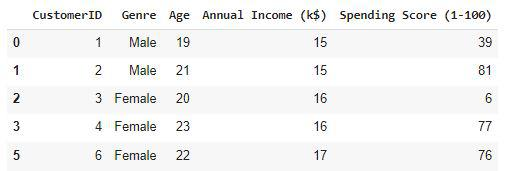First five rows after removing the 4th indexed row

This function can also be used to remove the columns of a data frame by adding the attribute axis =1 and providing the list of columns we would like to remove.

#### b) Renaming rows:

The rename function can be used to rename the rows or columns of the data frame.

## Python3

 `data_frame.rename({``0``:``"First"``,``1``:``"Second"``})`

Output: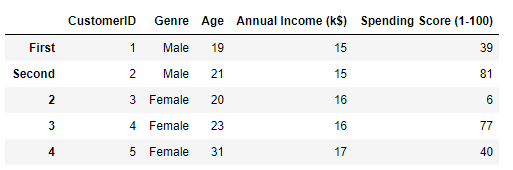Renamed rows of the data_frame

## Python3

 `#Creates a new column with all the values equal to 1` `data_frame[``'NewColumn'``] ``=` `1` `data_frame.head()`

Output: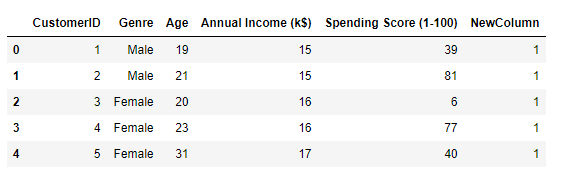Data frame with the new column

### Sorting DataFrame values:

#### a) Sort by column:

The sort_values( ) are the values of the column whose name is passed in the by attribute in the ascending order by default we can set this attribute to false to sort the array in the descending order.

## Python3

 `data_frame.sort_values(by``=``'Age'``, ascending``=``False``).head()`

Output: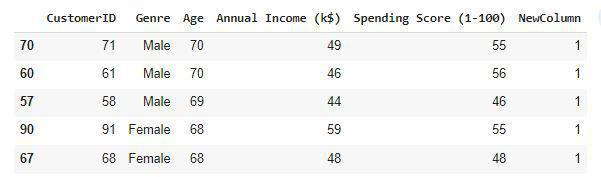Data frame with sorted age column values in descending order.

## Python3

 `data_frame.sort_values(by``=``[``'Age'``,``'Annual Income (k\$)'``]).head(``10``)`

Output: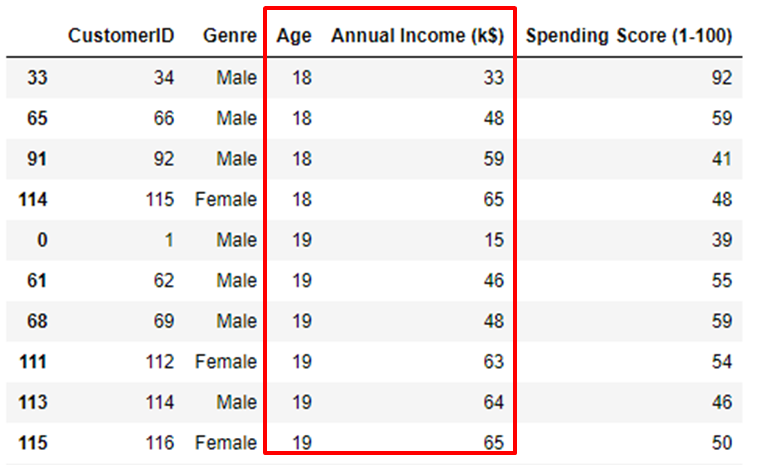Data frame sorted by ‘Age’ and ‘Annual Income’ column

### Merge Data Frames

The merge() function in pandas is used for all standard database join operations. Merge operation on data frames will join two data frames based on their common column values. Let’s create a data frame.

## Python3

 `#Creating dataframe1` `df1 ``=` `pd.DataFrame({` `        ``'Name'``:[``'Jeevan'``, ``'Raavan'``, ``'Geeta'``, ``'Bheem'``], ` `        ``'Age'``:[``25``, ``24``, ``52``, ``40``], ` `        ``'Qualification'``:[``'Msc'``, ``'MA'``, ``'MCA'``, ``'Phd'``]})` `df1`

Output: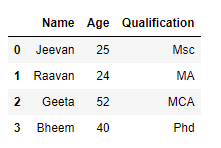Now we will create another data frame.

## Python3

 `#Creating dataframe2` `df2 ``=` `pd.DataFrame({``'Name'``:[``'Jeevan'``, ``'Raavan'``, ``'Geeta'``, ``'Bheem'``],` `                    ``'Salary'``:[``100000``, ``50000``, ``20000``, ``40000``]})` `df2`

Output: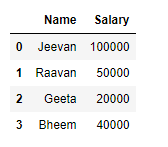Now. let’s merge these two data frames created earlier.

## Python3

 `#Merging two dataframes` `df ``=` `pd.merge(df1, df2)` `df`

Output: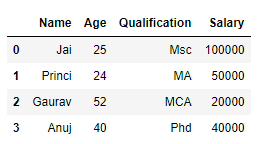Merged data frame

### Apply Function

a) By defining a function beforehand

The apply( ) function is used to iterate over a data frame. It can also be used with lambda functions.

## Python3

 `# Apply function` `def` `fun(value):` `    ``if` `value > ``70``:` `        ``return` `"Yes"` `    ``else``:` `        ``return` `"No"`   `data_frame[``'Customer Satisfaction'``] ``=` `data_frame[``'Spending Score (1-100)'``].``apply``(fun)` `data_frame.head(``10``)`

Output: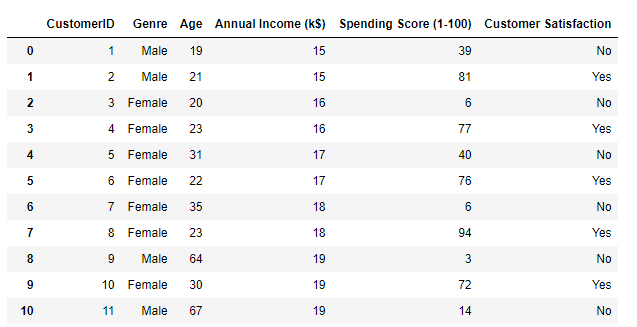The function applied to each row of the ‘Customer Satisfaction’ column

#### b). By using the lambda operator:

This syntax is generally used to apply log transformations and normalize the data to bring it in the range of 0 to 1 for particular columns of the data.

## Python3

 `const ``=` `data_frame[``'Age'``].``max``()` `data_frame[``'Age'``] ``=` `data_frame[``'Age'``].``apply``(``lambda` `x: x``/``const)` `data_frame.head()`

Output: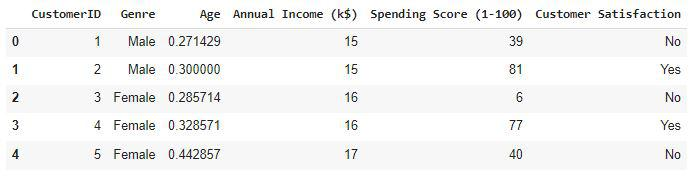First five rows after normalization

### Visualizing DataFrame

#### a) Scatter plot

The plot( ) function is used to make plots of the data frames.

## Python3

 `# Visualization` `data_frame.plot(x ``=``'CustomerID'``, y``=``'Spending Score (1-100)'``,kind ``=` `'scatter'``)`

Output: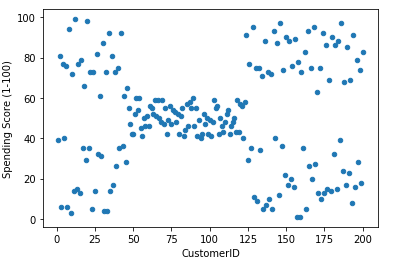Scatter plot of the Customer Satisfaction column

#### b) Histogram

The plot.hist( ) function is used to make plots of the data frames.

## Python3

 `data_frame.plot.hist()`

Output: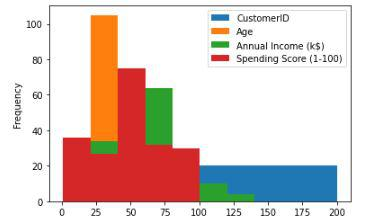Histogram for the distribution of the data

### Conclusion

There are other functions as well of pandas data frame but the above mentioned are some of the common ones generally used for handling large tabular data. One can refer to the pandas documentation as well to explore more about the functions mentioned above.

Whether you're preparing for your first job interview or aiming to upskill in this ever-evolving tech landscape, GeeksforGeeks Courses are your key to success. We provide top-quality content at affordable prices, all geared towards accelerating your growth in a time-bound manner. Join the millions we've already empowered, and we're here to do the same for you. Don't miss out - check it out now!